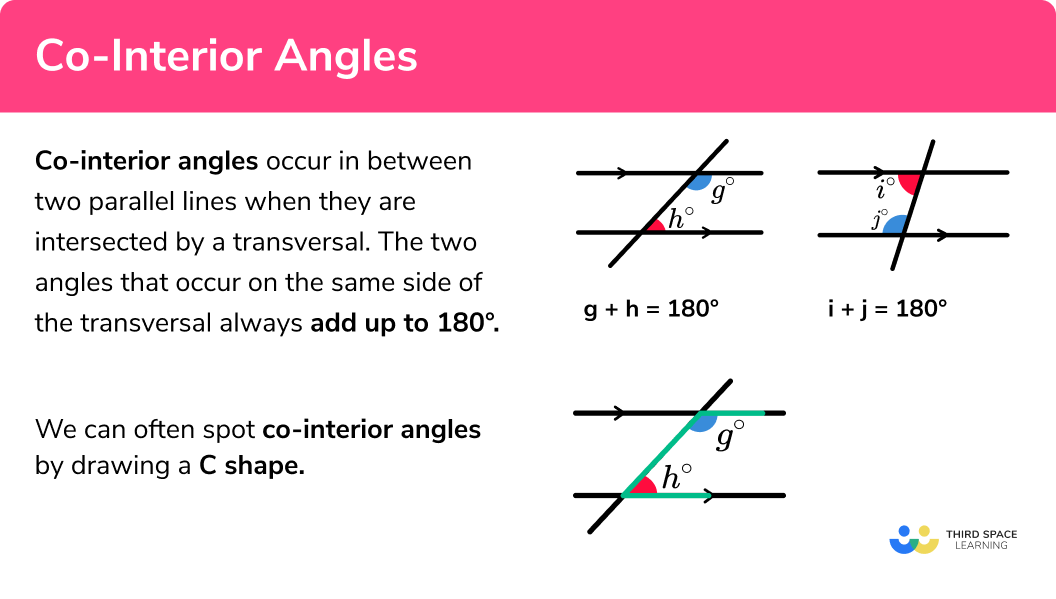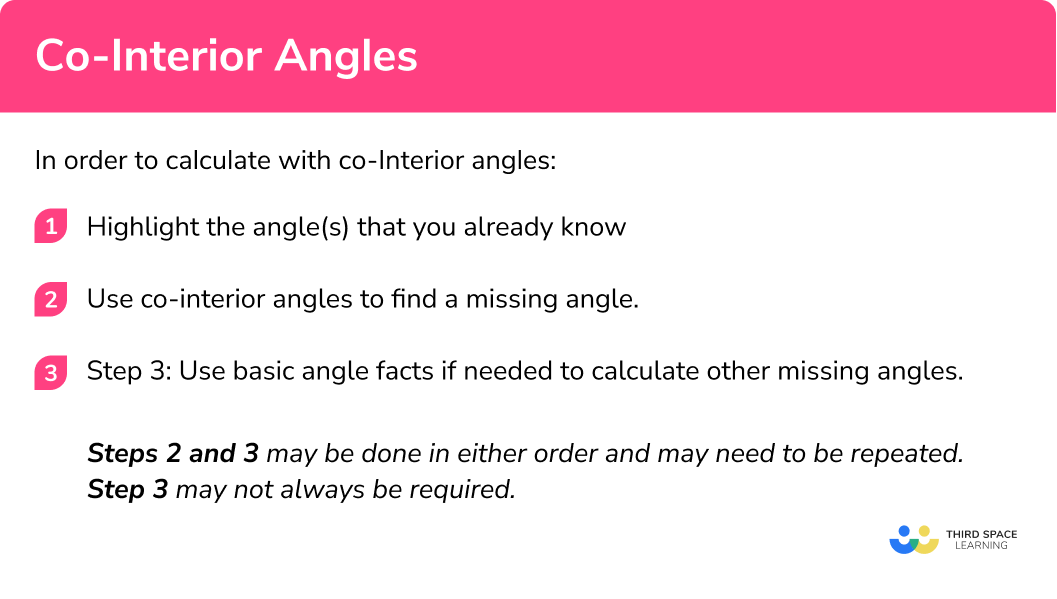# Co-Interior Angles

Here we will learn about co-interior angles including how to recognise co-interior angles, and apply this understanding to solve problems.

There are also angles in parallel lines worksheets based on Edexcel, AQA and OCR exam questions, along with further guidance on where to go next if you’re still stuck.

## What are co-interior angles?

Co-interior angles occur in between two parallel lines when they are intersected by a transversal. The two angles that occur on the same side of the transversal always add up to 180º.

Co-interior angles add up to 180ºg + h = 180^{\circ}i + j = 180^{\circ}k + l = 180^{\circ}m + n = 180^{\circ}

We can often spot co-interior angles by drawing a C shape.

The two interior angles are only equal when they are both 90º
In all other cases we can work out one of the co-interior angles by subtracting the other from 180º

### What are co-interior angles?## How to calculate with co-interior angles

In order to find a missing angle in parallel lines:

1. Highlight the angle(s) that you already know.
2. Use co-interior angles to find a missing angle.
3. Use basic angle facts to calculate the missing angle.

Steps 2 and 3 may be done in either order and may need to be repeated. Step 3 may not always be required.

### Explain how to calculate with co-interior angles## Angles in parallel lines – co-interior angles examples

### Example 1: co-interior angles

1. Highlight the angle(s) that you already know.

2Use co-interior angles to find a missing angle.

Here we can label the co-interior angle on the diagram as 60º as 120 + 60 = 180º.

3Use a basic angle fact to calculate the missing angle.

Here as θ is vertically opposite 60º,

θ = 60º.

### Example 2: co-interior angles

Here we can use either the 118º angle or the 83º angle. For this example we will use the 118º angle as this explores an alternative method not yet described.

As co-interior angles have a sum of 180º, 180 − 118 = 180º , the missing angle highlighted is 62º.

Here we have a trapezium with the sum of interior angles as 360º. Using this fact, we can calculate the value of θ:

\begin{aligned} \theta&=360^{\circ}-\left(118^{\circ}+62^{\circ}+83^{\circ}\right) \\\\ \theta&=97^{\circ} \end{aligned}

### Example 3: co-interior angles with algebra

We need to find the value of x first.

As 11x and 4x are co-interior, we can state that

\begin{aligned} 11x+4x&=180^{\circ} \\ 15x&=180^{\circ} \\ x&=12^{\circ} \end{aligned}

This gives us the two co-interior angles of 11x = 132º. and 4x = 48º.

The angles in the triangle are 48º and 90º, since the sum of angles in a triangle is 180º.

180 – 48 – 90 = 180º so the third angle in the triangle is 42º.

Since \theta and 42^o are on a straight line,

\begin{aligned} \theta&=180-42 \\\\ \theta&=138^{\circ} \end{aligned}

### Common misconceptions

• Mixing up angle facts

There are a lot of angle facts and it is easy to mistake alternate angles with corresponding angles. To prevent this from occurring, think about co-interior angles being inside the C shape.

• Using a protractor to measure an angle

Using a protractor to measure an angle. Most diagrams are not to scale and so using a protractor will not result in a correct answer unless it is a coincidence.

Co-interior angles is part of our series of lessons to support revision on angles in parallel lines. You may find it helpful to start with the main angles in parallel lines lesson for a summary of what to expect, or use the step by step guides below for further detail on individual topics. Other lessons in this series include:

### Practice co-interior angles questions\theta =136^{\circ}\theta =44^{\circ}\theta =143^{\circ}\theta =37^{\circ}\theta and 44^{\circ} are co-interior angles. Therefore,

\theta = 180-44=136^{\circ}

2. Calculate the value of \theta\theta =125^{\circ}\theta =55^{\circ}\theta =70^{\circ}\theta =62.5^{\circ}Using co-interior angles, we can calculate

180-125=55^{\circ}Using co-interior angles again, we can see that

\theta=180-55=125^{\circ}

3. Given the information in the diagram, calculate the size of angle \theta\theta =110^{\circ}\theta =70^{\circ}\theta =40^{\circ}\theta =140^{\circ}Using co-interior angles, we can calculate

180-110=70^{\circ}Since it is an isosceles triangle, the other angle at the bottom of the triangle is 70^{\circ} as well.

Then, using angles in a triangle,

\theta=180-(70+70)=40^{\circ}

4. Calculate the value of  \theta\theta =90^{\circ}\theta =95^{\circ}\theta =85^{\circ}\theta =83^{\circ}(7 + \theta)^{\circ} and 88^{\circ} are co-interior angles therefore\begin{aligned} (7+ \theta) +88&=180\\\\ \theta&=180-(88+7)\\\\ \theta&=85^{\circ} \end{aligned}

5. Work out the value of   \theta\theta =68^{\circ}\theta =44^{\circ}\theta =112^{\circ}\theta =22^{\circ}Since the triangle is an isosceles triangle, we know that the other angle at the top of the triangle is 68^{\circ}.

(\theta+68)^{\circ} and 68^{\circ} are co-interior angles therefore(\theta+68) + 68 = 100 \theta = 180-(68+68) \theta=44\degree

6. By calculating the value for x , work out the size of each angle labelled.42^{\circ}  and  38^{\circ}30^{\circ}  and  30^{\circ}80^{\circ}  and  100^{\circ}78^{\circ}  and  102^{\circ}3x+12 and 2x+18 are co-interior angles, and so add up to 180\degree.

Therefore, we can write

3x+12+2x+18=180

5x+30=180

5x=150

x=30
so

3x+12=3 \times 30 + 12=102\degree

2x+18=2 \times 30+18=78\degree

### Co-interior angles GCSE questions

1.(a) Find the size of angle x .

(b) Find the size of angle y .

(3 marks)

(a)

180-38=142^{\circ} as co-inteior angles add to 180^{\circ}

180-142=38^{\circ} as angles on a straight line add to 180^{\circ}

(2)

(b)

180 – {(110 + 38)}=32^{\circ}

(1)

2. Work out the value of x .(3 marks)

5x – 7 + 3x + 11 = 180

(1)

8x + 4 = 180

(1)

x=22^{\circ}

(1)

3. Are the lines AB and CD parallel? Give a reason for your answer.(2 marks)

No,

95+87=182^{\circ}

(1)

When two lines are parallel, the co-interior angles add up to 180^{\circ}

(1)

## Learning checklist:

You have now learned how to:

• Apply the properties of angles at a point, angles at a point on a straight line, vertically opposite angles
• Understand and use the relationship between parallel lines and alternate and corresponding angles

## Still stuck?

Prepare your KS4 students for maths GCSEs success with Third Space Learning. Weekly online one to one GCSE maths revision lessons delivered by expert maths tutors.

Find out more about our GCSE maths tuition programme.